﻿ Designing Machine Learning Method for Software Project Effort PredictionComputer Science and Engineering

p-ISSN: 2163-1484    e-ISSN: 2163-1492

2019;  9(1): 6-11

doi:10.5923/j.computer.20190901.02Designing Machine Learning Method for Software Project Effort Prediction

Ketema Kifle Gebretsadik1, Walelign Tewabe Sewunetie2

1Msc. In Software Engineering, Lecturer of Software Engineering at Debre Markos University, Ethiopia

2Ass. Professor in Computer Science, Lecturer of Information Technology at Debre Markos University, Ethiopia

Correspondence to: Ketema Kifle Gebretsadik, Msc. In Software Engineering, Lecturer of Software Engineering at Debre Markos University, Ethiopia.
 Email: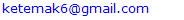Abstract

Software project effort Prediction is the most challenging and important activities in software project development. In software Industry when the size of the project and number of developers increases the project become complex, at this point accuracy prediction is strongly required during the early stages of project development. But to predict at early stages data and information are not available at the preliminary phases of project as well as the data is not complete, consistent and certain. In this research work we uses Artificial Neural network, Fuzzy logic, use case point model, modified environmental factor and revised use case point to predict software project effort at early stages. Artificial Neural Network has the ability to learn from previous data and fuzzy logic deals with uncertainty and also it provides a technique to deal with imprecision and information granularity. Also the modified environmental factor and revised use case point are used to determine the effort at early stage of the project development. In our experiment we compares each models, fitting accuracy using MMRE and PRED (0.25). The fitting accuracy of the models in terms of MMRE for Neuro-Fuzzy-UCBEM is 0.03, Neuro_UCBEM 0.13, Fuzzy-UCBEM 0.12 and UCBEM 0.22 and the fitting accuracy of the models in terms of Pred (0.25) for Neuro-Fuzzy-UCBEM is 1, Neuro_UCBEM 0.93, Fuzzy-UCBEM 0.93 and UCBEM 0.8. So our experiment result shows that Neuro-fuzzy logic model using revised use case point and modified environmental is best out performing model for software project development effort prediction at early stage than another models. Since the Neuro-fuzzy-UCBEM shows low value of MMRE (Mean of Magnitude of Relative Error) and high value prep (0.25) than Neuro-UCBEM, UCBEM and Fuzzy-UCBEM models.

Keywords: Machine Learning, Neural Network, Fuzzy Logic, Artificial Neural Network, Modified environmental factor, MMRE

Cite this paper: Ketema Kifle Gebretsadik, Walelign Tewabe Sewunetie, Designing Machine Learning Method for Software Project Effort Prediction, Computer Science and Engineering, Vol. 9 No. 1, 2019, pp. 6-11. doi: 10.5923/j.computer.20190901.02.

1. Introduction

Functional software project effort Prediction is one of the most challenging activities in software project development. Starting from the software development era until today, software development interest has become increasingly time to time but the predication approaches become more complex and expensive, particularly if it includes hundreds of people working over a long period of time. A project of this dimension can easily turn into disorder if proper management and controls are not in place. Software projects usually don’t fail during the implementation and most project fails are during the planning and estimation steps (Borade, 2013) due to going over time and cost.
They are a number of parameter for software project predictions. These are like Expert Judgment, COCOMO Model, Source Line of Code (SLC) and machine learning. There is a number parameters that affect the software project prediction, like a lack of data on completed software projects, lack of prediction of the staff’s skill level, Lack of understanding the requirements, improper software size estimation and Uncertainty of system and software requirements (Nassif A. B., 2013).

2. Software Project Effort Prediction Techniques

2.1. Use Case Point Model

The Use Case Point (UCP) model is based on mapping a use case diagram to a size metric called use-case points (Nassif A. B., " Software Size and Effort Estimation from Use Case Diagrams Using Regression and Soft Computing Models,", 2012). A use case diagram shows how users interact with the system and it is composed of Use Cases which represent the functional requirements and where an actor is a role played by a user. It is one of the Unified Modeling Language (UML) diagrams have become popular in the last decade (Nassif A. B., 2013), so it becomes more interested in conducting software project effort estimation by taking the use case diagrams.
The use case point model was first described by Karner (Jha, 2014). This model is used for software cost estimation based on the use case diagrams. The main components of the use case diagrams are actors and use cases. Use case point (UCP) is calculated from use case model. The general process in Use Case based prediction model involves the following major steps:
1. Unadjusted Actor Weight (UAW): In the UCP, actors are classified as simple, average or complex. The table 1 shows the actor classification according to the Karner (Karner, 1993) and newly added actor classification (Pragya Jha, 2014).
 Table 1. Actor Classification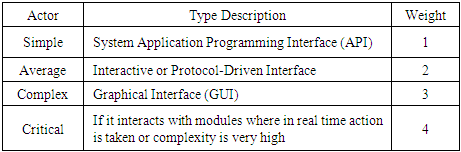The formula to calculate Unadjusted Actor Weight (UAW) according to (Karner, 1993) and (Pragya Jha, 2014).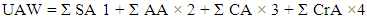(1)
Where SA, AA, CA and CrA correspond to Simple Actors, Average Actors, Complex Actors and Critical Actor respectively.
2. Unadjusted Use Case Weight (UUCW): Use cases are classified based on the number of transactions in the success and alternative scenarios (flows) and Included and extended use cases. The table 2 shows the Use Case classification according to the Karner (Karner, 1993) and newly added actor classification (Pragya Jha, 2014).
 Table 2. Use Case Classification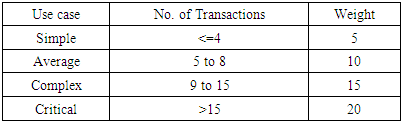The formula to calculate Unadjusted Use Case Weight (UUCW) according to (Karner, 1993) and (Pragya Jha, 2014).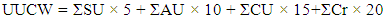(2)
Where SU, AU, CU and Cr correspond to Simple Use Case, Average Use Case, Complex Use Case and critical use case.
3. Unadjusted Use Case Points (UUCP): This is the summation of UAW with UUCW. This is described as: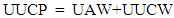(3)
According to and (Pragya Jha, 2014).
4. Technical Factor (TF): These factors contribute to the complexity of the project. The technical factors measure the complexity of a project regarding non-functional requirements.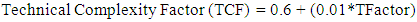(4)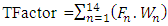(5)
Where Tfactor, Fn, Wn are technical factor, factor and weight value for n of this category respectively. The table 3 shows the Technical factor according to the Karner (Karner, 1993) and newly added Technical factor classification (Pragya Jha, 2014).
 Table 3. Technical factor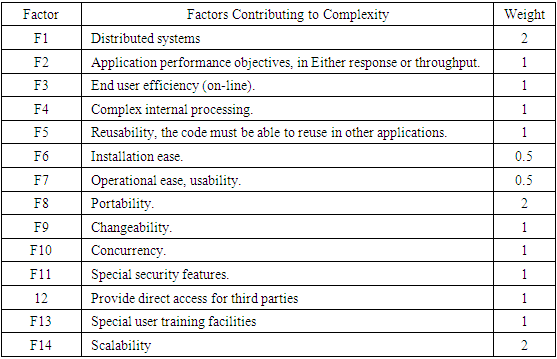5. Environmental Factor (EF): These factors contribute to the team efficiency and productivity.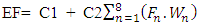(6)
Where C1 = 1.4, C2 = -0.03 and Fi is a factor which is equivalent to the Fi of the technical factor (i.e. between 0 and 5). The table 4 shows the Environmental factor according to the Karner (Karner, 1993) and newly added Environmental factor classification (Pragya Jha, 2014).
 Table 4. Environmental factor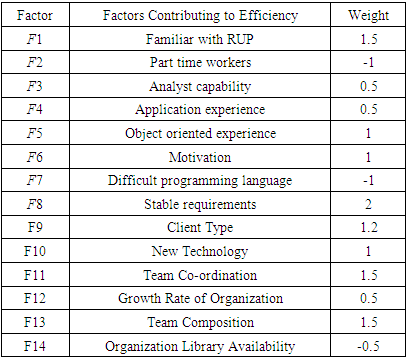6. Adjusted Use Case Points (UCP): The UCP is calculated by multiplying the UUCP by the technical and environmental factors as follows: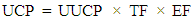(7)
7. Effort: This is the final stage of the use case point model. Karner proposed 20 person-hours for each UCP. This can be represented as (Nassif A. B., 2012) (Jha, 2014):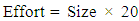(8)

2.2. Machine Learning Models

In this research work we use the hybrid machine learning approaches for software project effort prediction. In this paper we take the best features of the Articial Neural Network and Fuzzy Logic.
a. Artificial Neural Networks (ANN)
Artificial Neural Network (ANN) is a computational or mathematical method that is simulated by the biological human brain (Nassif A. B., " Software Size and Effort Estimation from Use Case Diagrams Using Regression and Soft Computing Models,", 2012). It have several layers each layer is composed of several elements called neurons. Neurons investigating the weights defined for inputs to produce the outputs. Outputs is the actual effort, which are the main goals of prediction (Khatibi V. a., 2011). Through learning process ANN can be configured for a specific application, such as pattern reorganization or data clarification. Back propagation neural network is the best selection for software prediction problem. Because it adjust the weight by comparing the network outputs and actual result (Tailor1, 2014) (Nassif A. B., 2013). In addition, training is done effectively. Artificial neural network are used in effort prediction due to its ability to learn from previous data (Attarzadeh I. a., January 2010). It is also able to model complex relationships between the dependent (effort) and independent variables (cost drivers). In addition, it has the ability to generalize from the training data set thus enabling it to produce acceptable result for previously unseen data.
Neural networks are nets of processing elements that are able to learn the mapping existent between input and output data. The neuron computes a weighted sum of its inputs and generates an output if the sum exceeds a certain threshold. This output then becomes an excitatory (positive) or inhibitory (negative) input to other neurons in the network. The process continues until one or more outputs are generated.
The Neural Network is initialized with random weights and gradually learns the relationships implicit in a training data set by adjusting its weights when presented to these data. The network generates effort by propagating the initial inputs through subsequent layers of processing elements to the final output layer. Each neuron in the network computes a nonlinear function of its inputs and passes the resultant value along its output. The favored activation function is Sigmoid Function (Sehr, 2011) (K., 2002) given as: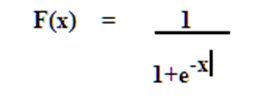Function 1. Sigmoid Function (K., 2002)
b. Fuzzy Logic (FL)
All system which works based on the fuzzy logic try to simulate human behavior and reasoning (Khatibi V. a., 2011). Fuzzy logic is a mathematical tool for dealing with uncertainty and also it provides a technique to deal with imprecision and information granularity.
A fuzzy set is a set with a smooth boundary (Lalitha. R.V.S, 2012) (Srinivasa Rao.T, December 2011). Fuzzy set theory generalizes classical set theory to allow partial membership (Ziauddin, 2012). The best way to introduce fuzzy sets is to start with a limitation of classical sets. A set in classical set theory always has a sharp boundary because membership in a set is a black-and-white concept, i.e. an object either completely belongs to the set or does not belongs to the set at all. The degree of membership in a set is expressed by a number between 0 and 1; 0 means entirely not in the set, 1 means completely in the set, and a number in between means partially in the set. This way a smooth and gradual transition from the region outside the set to those in the set can be described. A fuzzy set is thus defined by a function that maps objects in a domain of concern to their membership value in the set. Such a function is called the Membership Function and usually denoted by the Greek symbol μ. The membership function of a fuzzy set A is denoted by μA, and the membership value of x in A is denoted by μA(x). The domain of membership function, which is the domain of concern from which elements of the set are drawn, is called the Universe Of Discourse. We may identify meaningful lower and upper bounds of the membership functions. Membership functions of this type are known as interval values fuzzy sets. The intervals of the membership functions are also fuzzy then it is known as interval Type-2 fuzzy sets.
This research examines the potential of two soft Machine Learning Models and one algorithmic approach i.e. fuzzy logic and artificial neural networks and Use Case Point respectively for software project development effort estimation models.

3. Mixed Software Project Prediction Models

3.1. Fuzzy Logic Approach with Use Case Based Effort Estimation Model

A fuzzy set is represented by a membership function. Each element will have a grade of membership that represents the degree to which a specific element belongs to the set.
The central idea of extending Use Case Based Estimation Model (UCBEM) to Fuzzy -UCBEM through fuzzy set theory is to expand semantics (Simple, Average, and Complex) used to categorize use case complexities.
A triangular membership fuzzy number can be represented by A (a, b, c), whose membership functions are presented in the following equation (Divya Kashyap, 2014):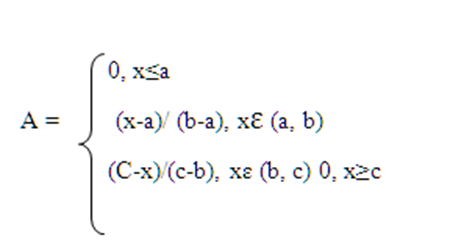Equation 2. Triangular Membership
The values a, b, and c respectively identify the lower, middle, and upper limits that determine the shape of the triangle.

3.2. Neural Network Approach with Use Case Based Effort Estimation Model

The actual weight calculation also includes the slope of the filtering (activation) function and a learning rate value. The favored function for the back-propagation algorithm is the sigmoid function (Attarzadeh I. &., 2010). Since the function is not linear, it is appropriate for software effort estimation problem.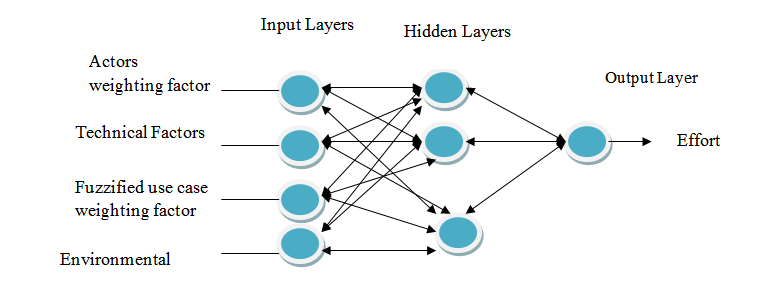Figure 1. A Neural Network for Software Project Development Effort Estimation

3.3. Neuro-Fuzzy with Use Case Based Effort Estimation Model

For Neuro-Fuzzy use Case Based experiment input data (variables) are collected first. The input variables selected for the neural network model are based on the use case based effort estimation model. These variables are
1. Actor’s complexity weighting factors (AW)
2. Use case complexity weighting factors (Fuzzified use case complexity weighting factors in the case of Fuzzy-UCBEM)-UCW.
3. Technical complexity weighting factors (TCF)
4. Environmental factors (EF)

4. Model Evaluation Approach

In this work, In order to evaluate the techniques with respect to their fitting accuracy, Relative Error (RE), Magnitude of Relative Error (MRE) and Mean Magnitude of Relative Error (MMRE) and Prediction at Level P (Prep(P) are used, where P is a percentage needed. We use this methods because MMRE and PRED are the most widely used metrics for evaluating the accuracy of cost estimation models (Nguyen, 2010).
The Relative Error (RE) and Magnitude of Relative Error (MRE) (Attarzadeh I. a., January 2010) (Iraji, 2012) (Wei Lin Du, 2010) which is defined as follows.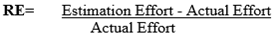The RE is used to calculate the estimation accuracy
Magnitude of Relative Error (MRE) is defined as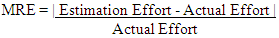Or Magnitude of Relative Error (MRE) is defined as (Reddy, 2009) (Raju, 2010)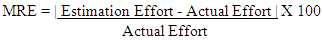Mean Magnitude of Relative Error (MMRE)
The mean magnitude of relative error (MMRE) is the average of all magnitudes of relative errors. MMRE is defined as follows (Ali Idri, 2004):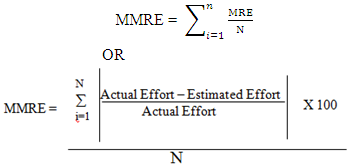Where N is the number of projects
MMRE: This is a very common criterion used to evaluate software cost estimation models (Nassif A. B., 2012).
Prediction Level (PRED)
PRED (P) =k/n
Where P is the maximum MRE of a selected range, n is the total number of projects, and k is number of projects in a set of n projects whose MRE <= P (k is the number of observations with a MRE less than or equal to p). A common value for p is 0.25 (Ali Idri, 2004) (Idri, 2002). PRED calculates the ratio of projects’ MREs that falls into the selected range (P) out of the total projects.
A standard criteria for considering the model as acceptable is Prep (0.25) ≥ 0.75 (Malathi, 2012) (B.A. Kitchenham, 2001). This means that at least 75% of the estimate is within the range of 25% of actual values. A model which gives higher Prep (0.25) is a better model.

5. Discussion of the Result

In order to evaluate the techniques with respect to their fitting accuracy, Mean Magnitude of Relative Error (MMRE) and Prep (I) methods were used. These methods are accepted as common accuracy indicators of software cost estimation models (Nassif A. B., 2012). They are also considered as more reasonable variables than other statistical criteria, since they measure the capability of prediction, not the statistical explanation. The relative error (RE) is ((actual effort-estimated effort) / actual effort) *100. The magnitude of relative error (MRE) is the absolute value of the relative error (MRE=|RE|). The mean magnitude of relative error (MMRE) is the average of all magnitudes of relative errors. MMRE is defined as follows: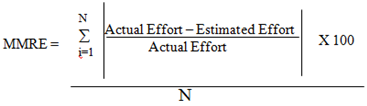Where N is the number of projects
The most widely used evaluation criterion to assess the performance of software effort estimation models is the Mean Magnitude of Relative Error (MMRE), when MMRE is used in the evaluation, good results are implied by lower values of MMRE or If the MMRE is small, then we have a good set of Predictions (Nassif A. B., 2010). A model is accepted as a good effort estimation model, if it estimates with MMRE ≤ 0.25 (Foss, 2003).
The accuracy of UCBEM, Fuzzy_UCBEM, Neuro-Fuzzy-UCBEM and Fuzzy-UCBEM in terms of the above criteria’s is computed, and the result is shown in the following table 5.
 Table 5. Summary of results in terms of MMRE and PRED (0.25)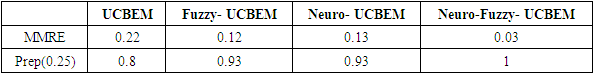The experimentation results in terms of both MMRE and Prep (0.25) shows that the Neuro-Fuzzy-UCBEM technique appears as best outperform model than Fuzzy-UCBEM, Neuro-UCBEM and UCBEM models. The result indicates that the neural network model out performs best when it is used with fuzzy use case complexity weighting factor inputs, and it is to some extent better than UCBEM when it is used the fuzzyfing inputs.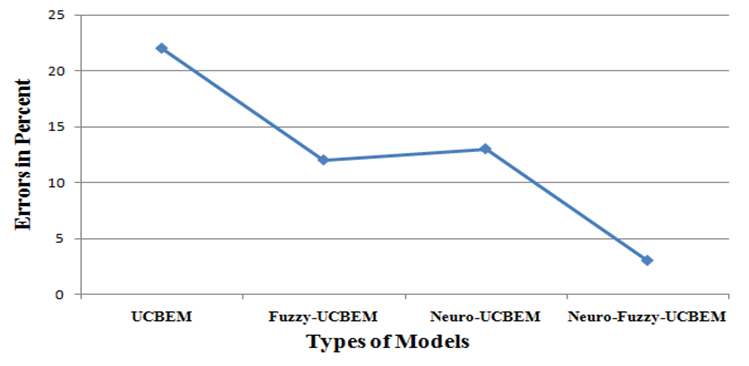Figure 2. Performance of models in terms of MMRE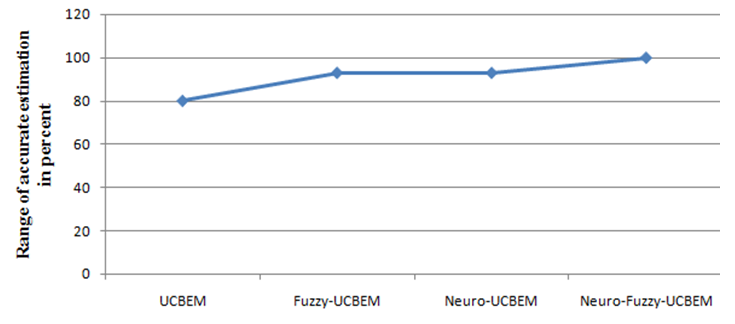Figure 3. Performance of models in terms of Prep (0.25)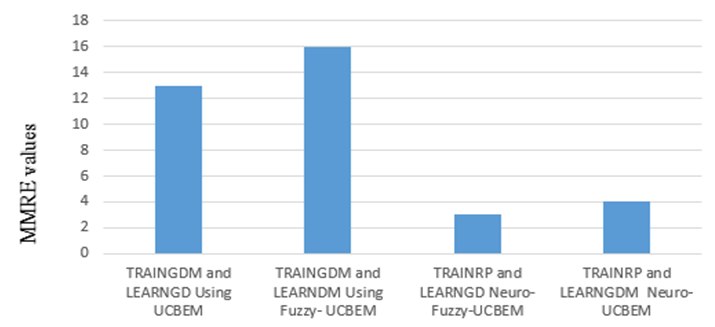Figure 4. Models with training and learning function using MMRE value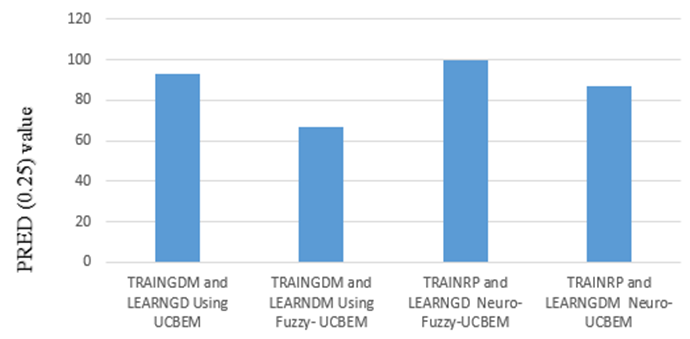Figure 5. Models with training and learning function using PRED (0.25) value
As we have seen in the above graph TRAINRP and LEARNGD functions shows small percentage of MMRE value than the other three training and learning functions. So the small number of MMRE value is best estimation model indicator (Foss, 2003).

6. Conclusions and Future Works

In this research work we have tried to address potential issue of machine learning approaches like neural network, fuzzy logic and modified environmental factor with the use case point for software project effort prediction. So we believe that the potential of machine learning approaches and Revised Use Case Point (Re-UCP) could be further explored by adding more historical data and one can come up with better results by extending this work. So in the future we have planned to work Using Revised Use Case Point (Re-UCP) method for more reliable data set. Compering the extended use point and Revised Use Case Point (Re-UCP) method using Neuro-fuzzy methods. Adding environmental factor and technical factor. It is also possible to check the performance of the neural network model with other training and transfer functions.

References

  Ali Idri, A. A. (2004). Validating and Understanding Software Cost Estimation Models based on Neural Networks.  Attarzadeh, I. &. (2010). "Soft Computing Approach for Software Cost Estimation," .  Attarzadeh, I. a. (January 2010). "Soft Computing Approach for Software Cost Estimation," .  Attarzadeh, I. a. (January 2010). Soft Computing Approach for Software Cost Estimation.  B.A. Kitchenham, L. S. (2001). What accuracy statistics really measure.  Borade, J. G. (2013). Software Project Effort and Cost Estimation Techniques. Volume 3.  Divya Kashyap, D. S. (2014). Refining the Use Case Classification for use Case Point Method for Software Effort Estimation.  Foss, T. S. (2003). A simulation study of the model evaluation criterion MMRE. Software Engineering. IEEE Transactions on, 29(11), 985-995.  Idri, A. K. (2002). Can neural networks be easily interpreted in software cost estimation? IEEE.  Iraji, M. S. (2012). Object Oriented Software Effort Estimate with Adaptive Neuro Fuzzy use Case Size Point.  Jha, P. P. (2014, December). "Estimating Software Development Effort using UML Use Case Point (UCP) Method with a Modified set of Environmental Factors," Diploma, University of Linkoping.  K., T. M. ( 2002). " Can neural networks be easily interpreted in software cost estimation?,".  Karner, G. (1993, December). Metrics for objectory. Diploma, University of Linkoping.  Khatibi, V. a. ( 2011). "Software Cost Estimation Methods: A Review,".  Khatibi, V. a. (2011). Software Cost Estimation Methods: A Review.  Lalitha.R.V.S. (2012). MARKOV MODEL: Analyzing its behavior for Uncertainty conditions. Vol 2.  Malathi, S. &. (2012). Analysis Of Size Metrics And Effort Performance Criterion In Software Cost Estimation.  Nassif, A. B. (2013). "Towards an early software estimation using log-linear regression and a multilayer perceptron model,".  Nassif, A. B. (2010). Enhancing use case points estimation method using soft computing techniques. Journal of Global Research in Computer Science, 1(4).  Nassif, A. B. (2012). Software Size and Effort Estimation from Use Case Diagrams Using Regression and Soft Computing Models.  Nassif, A. B. (2012). " Software Size and Effort Estimation from Use Case Diagrams Using Regression and Soft Computing Models," .  Nassif, A. B. (2013). Towards an early software estimation using log-linear regression and a multilayer perceptron model.  Nguyen, V. (2010). Improved Size and Effort Estimation Models For Software Maintenance.  Pragya Jha, P. P. (2014). Estimating Software Development Effort using UML Use Case Point (UCP) Method with a Modified set of Environmental Factors.  Raju, R. a. (2010). An Optimal Neural Network Model for Software Effort Estimation.  Reddy, C. S. (2009). A concise neural network model for estimating software effort.  Sehr, Y. S. (2011). "Soft Computing Techniques For Software Project Effort Estimation," . (3).  Srinivasa Rao.T, P. R. (December 2011). Fuzzy and Swarm Intelligence for Software Cost Estimation. Volume 11(Issue 22).  Tailor1, J. S. (2014). "Comparative Analysis Of Software Cost And Effort estimation Methods: A Review,".  Wei Lin Du, D. H. (2010). Improving Software Effort Estimation Using Neuro-Fuzzy Model with SEER-SEM.  Ziauddin, S. K. (2012). Software Cost Estimation Using Soft Computing Techniques. Vol. 2(1).